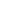# Free CBSE Class 9 Mathematics Unit 2-Algebra Linear equations in 2 variables Worksheets

Download free printable Linear equations in 2 variables Worksheets to practice. With thousands of questions available, you can generate as many Linear equations in 2 variables Worksheets as you want.

## Sample CBSE Class 9 Mathematics Unit 2-Algebra Linear equations in 2 variables Worksheet Questions

1.

Which of the following linear equations is suitable for the graph given below ?1. y + x = 7 2. x - y = 7 3. -x - y = 7 4. x - 2y = 7
2.

The area of the triangle formed by intersection ofx- axis , y-axis and the line shown in given figure is:3.

Equation of the represented by following graph is:1. y = x 2. x + y = 0 3. x + 2y = 0 4. x = 0
4.

The slope of following graph is:5.

Equation of the represented by following graph is:1. 3x -5y = 30 2. 10x -5y = 30 3. -3x -5y = 30 4. 3x +5y = 30
6.

Equation of the represented by following graph is:1. y = 6 2. x = -6 3. y + 6 = 0 4. x = 6
7.

Equation of the represented by following graph is:1. 2x - y = 7 2. 2x + y = 7 3. x - 2y = 7 4. 2x - y = - 7
8.

The following graph is represented by which two linear equation1. 2x + y = 3 and 2x â?? y + 2 = 0 2. 2x - y = 6 and 4x â?? y + 2 = 0 3. 2x + 5y = 6 and 2x â?? 3y + 2 = 0 4. 2x + y = 6 and 2x â?? y + 2 = 0
9.

Equation of the represented by following graph is:1. x = 3 2. x + y = 3 3. y + 3 = 0 4. y - 3 = 0
10.

The graphs of the equations x – y = 1 and 2x + y = 8 is given below  Determine the area bounded by these two lines y – axis.1. 16 sq unit 2. 15.5 sq unit 3. 13.5 sq unit 4. 10 sq unit

Worksheets by UrbanPro

Our worksheets are designed to help students explore various topics, practice skills and enrich their subject knowledge, to improve their academic performance. Designed by Experts who have extensive experience and expertise in teaching a subject, these worksheets will improve your child's problem-solving skills and subject knowledge in a fun and interactive manner.
Check out our free customized worksheets across school boards, grades, subjects and levels of subject knowledge. You can download, print and share these worksheets with anyone, anywhere, anytime!

Get a custom worksheet to practice!

Select your topic & see the magic.

subjectSelect Chapter(s)

Chapters & Subtopics